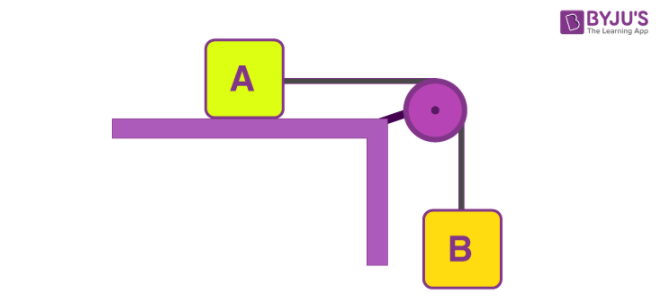# A block A of mass 7 kg is placed on a frictionless table A thread tied to it passes over a frictionless pulley and carries a body B of mass 3 kg at the other end. The acceleration of the system is (given g = 10 ms^-2) (a) 100 ms^-2 (b) 3 ms^-2 (c) 10 ms^-2 (d) 30 ms^-2For a body A

Tension = Mass x Acceleration

Let us consider Acceleration = a

Tension = ma = 7a

Fo a body B

Force = mass x acceleration

Ma = mg – T

3a = 3 x 10 – 7a

On calculating further, we get,

3a + 7a = 30

10a = 30

a = 30/10

We get,

a = 3 m/s2

Therefore, the correct option is (b)(0)(0)